# High School Math : How to find an angle in a right triangle

## Example Questions

### Example Question #1 : How To Find An Angle In A Right Triangle

Acute angles x and y are inside a right triangle. If x is four less than one third of 21, what is y?

Possible Answers:

18

3

7

87

90

Correct answer:

87

Explanation:

We know that the sum of all the angles must be 180 and we already know one angle is 90, leaving the sum of x and y to be 90.

Solve for x to find y.

One third of 21 is 7. Four less than  7 is 3. So if angle x is 3 then that leaves 87 for angle y.

### Example Question #2 : How To Find An Angle In A Right Triangle

If a right triangle has one leg with a length of 4 and a hypotenuse with a length of 8, what is the measure of the angle between the hypotenuse and its other leg?

Possible Answers:

60

45

90

30

65

Correct answer:

30

Explanation:

The first thing to notice is that this is a 30o:60o:90o triangle. If you draw a diagram, it is easier to see that the angle that is asked for corresponds to the side with a length of 4. This will be the smallest angle. The correct answer is 30.

### Example Question #3 : How To Find An Angle In A Right Triangle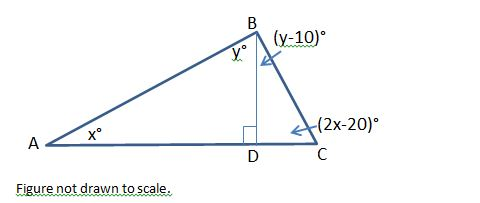In the figure above, what is the positive difference, in degrees, between the measures of angle ACB and angle CBD?

Possible Answers:

20

40

10

50

30

Correct answer:

10

Explanation:

In the figure above, angle ADB is a right angle. Because side AC is a straight line, angle CDB must also be a right angle.

Let’s examine triangle ADB. The sum of the measures of the three angles must be 180 degrees, and we know that angle ADB must be 90 degrees, since it is a right angle. We can now set up the following equation.

x + y + 90 = 180

Subtract 90 from both sides.

x + y = 90

Next, we will look at triangle CDB. We know that angle CDB is also 90 degrees, so we will write the following equation:

y – 10 + 2x – 20 + 90 = 180

y + 2x + 60 = 180

Subtract 60 from both sides.

y + 2x = 120

We have a system of equations consisting of x + y = 90 and y + 2x = 120. We can solve this system by solving one equation in terms of x and then substituting this value into the second equation. Let’s solve for y in the equation x + y = 90.

x + y = 90

Subtract x from both sides.

y = 90 – x

Next, we can substitute 90 – x into the equation y + 2x = 120.

(90 – x) + 2x = 120

90 + x = 120

x = 120 – 90 = 30

x = 30

Since y = 90 – x, y = 90 – 30 = 60.

The question ultimately asks us to find the positive difference between the measures of ACB and CBD. The measure of ACB = 2x – 20 = 2(30) – 20 = 40 degrees. The measure of CBD = y – 10 = 60 – 10 = 50 degrees. The positive difference between 50 degrees and 40 degrees is 10.

The answer is 10.

### Example Question #1 : How To Find An Angle In A Right Triangle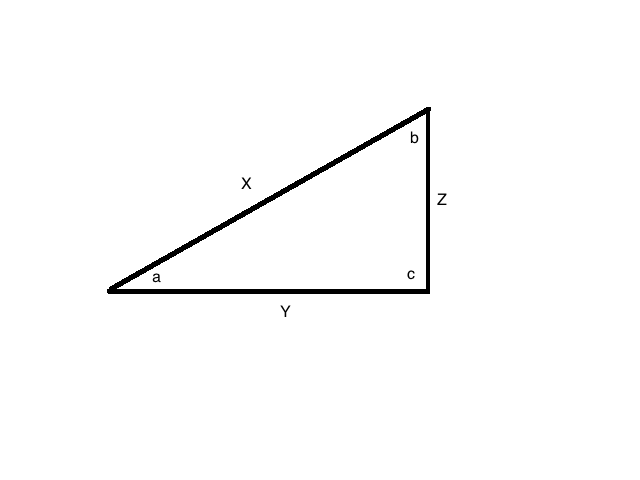If angle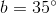and angle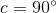, what is the value for angle?

Possible Answers: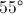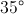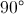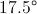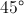Correct answer:Explanation:

For this problem, remember that the sum of the degrees in a triangle is.

That means that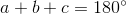.

Plug in our given values to solve: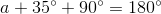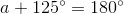Subtract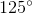from both sides: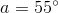### Example Question #4 : How To Find An Angle In A Right Triangle

Which of the following sets of line-segment lengths can form a triangle?

Possible Answers: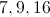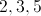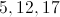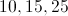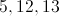Correct answer:Explanation:

In any given triangle, the sum of any two sides is greater than the third.  The incorrect answers have the sum of two sides equal to the third.

### Example Question #1 : How To Find An Angle In A Right Triangle

In right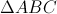,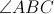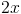and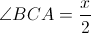.

What is the value of?

Possible Answers:

24

36

32

48

30

Correct answer:

36

Explanation:

There are 180 degrees in every triangle. Since this triangle is a right triangle, one of the angles measures 90 degrees.

Therefore,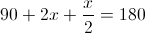.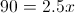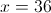### All High School Math Resources